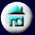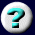11. Conservation of Mass,       Charge, and Energy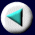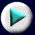Mass and Energy: Nuclear Reactions
 (We must include electrons in this calculation because 4.0026 amu is the mass of the helium-4 atom, not the nucleus.) This missing mass corresponds to 0.0305 x 931.4 MeV = 28.4 MeV of energy. If we could put together a helium atom directly from two neutrons, two protons, and two electrons, then 28.4 MeV of energy would be given off for every atom formed: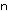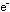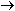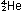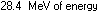Compared to common chemical reactions, this is an enormous quantity of energy. Since 1 electron volt per atom is equivalent to 23.06 kcal per mole,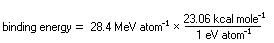= 655,000,000 kcal mole-1
Page 22 of 37